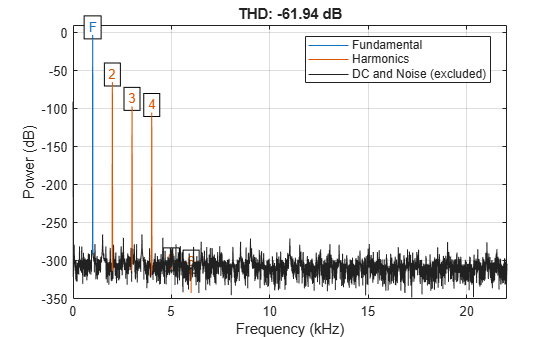# Measure Total Harmonic Distortion

This example shows how to measure the total harmonic distortion (THD) of a sinusoidal signal. The example uses the following scenario: A manufacturer of audio speakers claims the model A speaker produces less than 0.09% harmonic distortion at 1 kHz with a 1 volt input. The harmonic distortion is measured with respect to the fundamental (THD-F).

Assume you record the following data obtained by driving the speaker with a 1 kHz tone at 1 volt. The data is sampled at 44.1 kHz for analysis.

```Fs = 44.1e3; t = 0:1/Fs:1; x = cos(2*pi*1000*t)+8e-4*sin(2*pi*2000*t)+2e-5*cos(2*pi*3000*t-pi/4)+... 8e-6*sin(2*pi*4000*t);```

Obtain the total harmonic distortion of the input signal in dB. Specify that six harmonics are used in calculating the THD. This includes the fundamental frequency of 1 kHz. Input the sampling frequency of 44.1 kHz. Determine the frequencies of the harmonics and their power estimates.

```nharm = 6; [thd_db,harmpow,harmfreq] = thd(x,Fs,nharm);```

The function `thd` outputs the total harmonic distortion in dB. Convert the measurement from dB to a percentage to compare the value against the manufacturer's claims.

`percent_thd = 100*(10^(thd_db/20))`
```percent_thd = 0.0800 ```

The value you obtain indicates that the manufacturer's claims about the THD for speaker model A are correct.

You can obtain further insight by examining the power (dB) of the individual harmonics.

`T = table(harmfreq,harmpow,'VariableNames',{'Frequency','Power'})`
```T=6×2 table Frequency Power _________ _______ 1000 -3.0103 2000 -64.949 3000 -96.99 4000 -104.95 4997.9 -306.11 5998.9 -310.61 ```

The total harmonic distortion is approximately $-62$ dB. If you examine the power of the individual harmonics, you see that the major contribution comes from the harmonic at 2 kHz. The power at 2 kHz is approximately 62 dB below the power of the fundamental. The remaining harmonics do not contribute significantly to the total harmonic distortion. Additionally, the synthesized signal contains only four harmonics, including the fundamental. This is confirmed by the table, which shows a large power reduction after 4 kHz. Therefore, repeating the calculation with only four harmonics does not change the total harmonic distortion significantly.

Plot the signal spectrum, display the total harmonic distortion on the figure title, and annotate the harmonics.

`thd(x,Fs,nharm);`